Trang chủ / Toán Kangaroo / Lớp 7-8

Đề thi toán Kangaroo Canada lớp 7-8 năm 2011

1. Part A: Each correct answer is worth 3 points.

How many hours are there in half of a third of a quarter of a day?

2. The diagram shows a cube with sides of length 12 cm. An ant is walking across the cube’s surface from A to G using the path shown in bold. How far will it have to walk?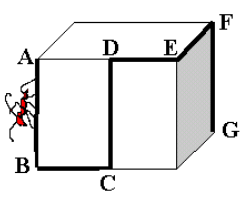3. In triangle ABC, the angle at A is three times greater than the angle at B and half of the angle at C. What is the angle at A?

4. There were 5 parrots in the cage. Their average price was 6000 dollars. One day during the cleaning of the cage the most beautiful parrot flew away. The average price of the remaining four parrots was 5000 dollars. What was the price of the parrot that escaped?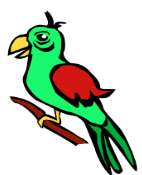5. A straight line is drawn across a 4×4 chessboard. What is the greatest number of 1×1 squares this line can pass through?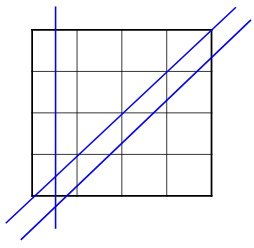6. In a triangle ABC the angle C is three times bigger then the angle A, the angle B is two times bigger then the angle A. Then the triangle ABC:

7. At my school, 50% of students swim. Of those, 30% are on the swim team. What percent of students at my school are on the swim team?

8. Which of the following numbers is odd for every integer n ?

9. In the picture, strips 1, 2 and 3 have the same horizontal width a. These strips connect two parallel lines. Which strip has the largest area?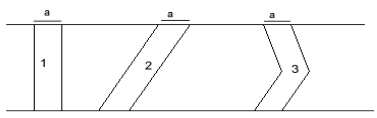10. A is the number 11111...1111 formed by writing 2003 ones in a row. What is the sum of the digits of the product 2003 x A?

11. When a barrel is 30% empty it contains 30 litres more than when it is 30% full. How many litres does the barrel hold when full?

12. In the picture there are four overlapping squares with sides 11, 9, 7 and 5 cm long. How much greater is the sum of the two grey areas than the sum of the two black areas?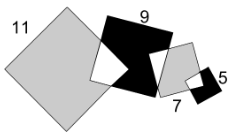13. What is the sum of the 10 angles in the picture?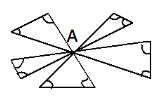14. Charles tells the truth every other day; otherwise he lies. Today he stated exactly four of the following statements. Which one could he not have stated today?

15. You have six sticks of lengths 1 cm, 2 cm, 3 cm, 2001 cm, 2002 cm and 2003 cm. You have to choose three of these sticks to form a triangle. How many different choices of three sticks are there that work?